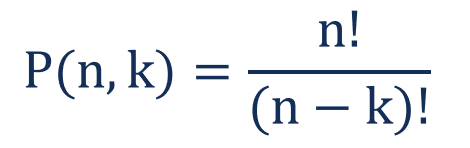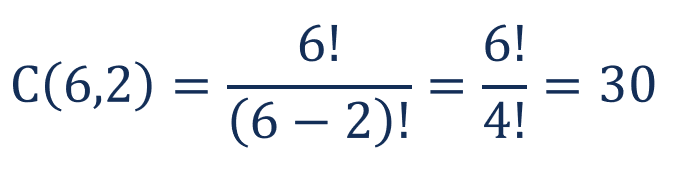# Permutation

A mathematical technique that determines the number of possible arrangements in a set when the order matters

## What is a Permutation?

A permutation is a mathematical technique that determines the number of possible arrangements in a set when the order of the arrangements matters. Common mathematical problems involve choosing only several items from a set of items with a certain order.Permutations are frequently confused with another mathematical technique called combinations. However, in combinations, the order of the chosen items does not influence the selection. In other words, the arrangements ab and be in permutations are considered different arrangements while in combinations, these arrangements are equal.

### Formula for Calculating Permutations

The general permutation formula is expressed in the following way:Where:

• n – the total number of elements in a set
• k – the number of selected elements arranged in a specific order
• ! – factorial

Factorial (noted as “!”) is the product of all positive integers less than or equal to the number preceding the factorial sign. For example, 3! = 1 x 2 x 3 = 6.

The formula above is used in situations when we want to select only several elements from a set of elements and arrange the selected elements in a special order.

### Example of a Permutation

You are a partner in a private equity firm. You want to invest \$5 million in two projects. Instead of equal allocation, you decided to invest \$3 million in the most promising project and \$2 million in the less promising project. Your analysts shortlisted six projects for potential investment. How many possible arrangements are available for your investment decision?

The example above is a permutation problem. Since the allocation of the money for the two projects is not equal, the selection order matters in this problem. For example, consider the following arrangement: invest \$3 million in Project A and \$2 million in Project B vs. invest \$2 million in Project A and \$3 million in Project B. The options are not equal with each other. Therefore, we must use the formula above to determine the number of available arrangements:Using the formula above, we can determine the number of available arrangements:Therefore, you can get 30 possible investment arrangements based on the six projects shortlisted by your analysts.